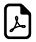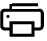Fraction Percentage Decimals Equivalents Chart & TableFraction,Percentage,Decimals Equivalents Conversion Chart

An online fraction-percentage-decimals conversion table and chart.Fraction Percentage Decimal
1/100 1% 0.01
1/50 2% 0.02
1/40 2½% 0.025
1/25 4% 0.04
1/20 5% 0.05
1/16 61/4% 0.0625
1/10 10% 0.1
1/8 12½% 0.125
3/8 37½% 0.375
5/8 62½% 0.625
7/8 87½% 0.875
1/5 20% 0.2
2/5 40% 0.4
3/5 60% 0.6
4/5 80% 0.8
1/4 25% 0.25
3/4 75% 0.75
1/3 331/3% 0.333
1/2 50% 0.5
9/10 90% 0.9
99/100 99% 0.99
100% 1
5/4 125% 1.25
3/2 150% 1.5
200% 2

FractionDecimal
1/3.3333
2/3.6667

FractionDecimal
1/5.2
2/5.4
3/5.6
4/5.8

FractionDecimal
1/7.1429
2/7.2857
3/7.4286
4/7.5714
5/7.7143
6/7.8571

FractionDecimal
1/8.125
3/8.375
5/8.625
7/8.875

FractionDecimal
1/9.1111
2/9.2222
4/9.4444
5/9.5556
7/9.7778
8/9.8889

FractionDecimal
1/12.0833
5/12.4167
7/12.5833
11/12.9167

FractionDecimal
1/16.0625
3/16.1875
5/16.3125
7/16.4375
9/16.5625
11/16.6875
13/16.8125
15/16.9375

Fraction = Percent = Decimals Equivalents

 1/2 = 50% = 0.5 1/3 = 33 1/3% = 0.3333 2/3 = 66 2/3% = 0.6666 1/4 = 25% = 0.25 3/4 = 75% = 0.75 1/5 = 20% = 0.2 2/5 = 40% = 0.4 3/5 = 60% = 0.6 4/5 = 80% = 0.8 1/6 = 16 2/3% = 0.1666 5/6 = 83 1/3% = 0.8333 1/7 = 14 2/7% = 0.142857 2/7 = 28 4/7% = 0.285714 3/7 = 42 6/7% = 0.428571 4/7 = 57 1/7% = 0.571428 5/7 = 71 3/7% = 0.714285 6/7 = 85 5/7% = 0.857142 1/8 = 12 1/2% = 0.125 3/8 = 37 1/2% = 0.375 5/8 = 62 1/2% = 0.625 7/8 = 87 1/2% = 0.875 1/9 = 11 1/9% = 0.111 2/9 = 22 2/9% = 0.222 4/9 = 44 4/9% = 0.444 5/9 = 55 5/9% = 0.555 7/9 = 77 7/9% = 0.777 8/9 = 88 8/9% = 0.888 1/10 = 10% = 0.1 3/10 = 30% = 0.3 7/10 = 70% = 0.7 9/10 = 90% = 0.9 1/11 = 9 1/11% = 0.090909 2/11 = 18 2/11% = 0.181818 3/11 = 27 3/11% = 0.272727 4/11 = 36 4/11% = 0.363636 1/12 = 8 1/3% = 0.08333Math FormulasAge Calculators ►

Horse Age Calculator , Cow Age Calculator , Cat Age Calculator , Dog Age Calculator, Human Age Calculator 100 Days Old Calculator Date Difference Calculator